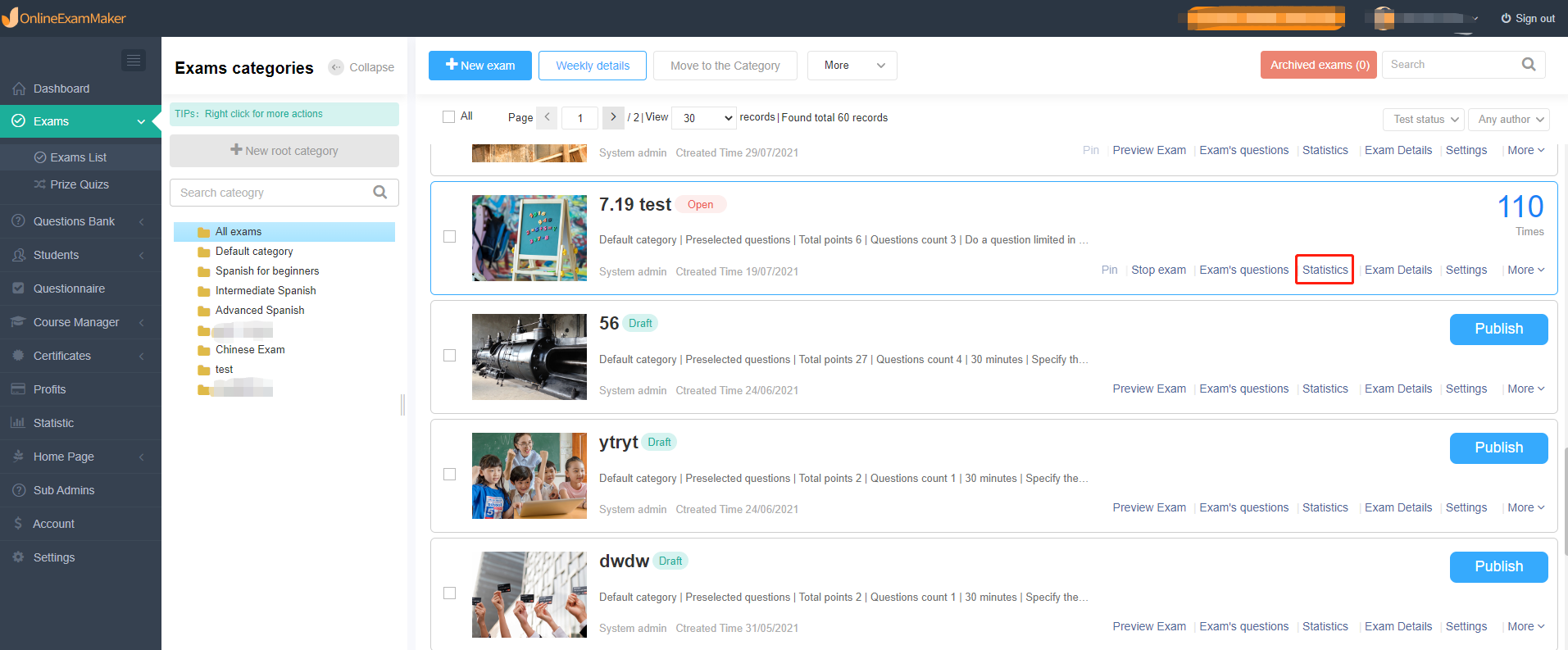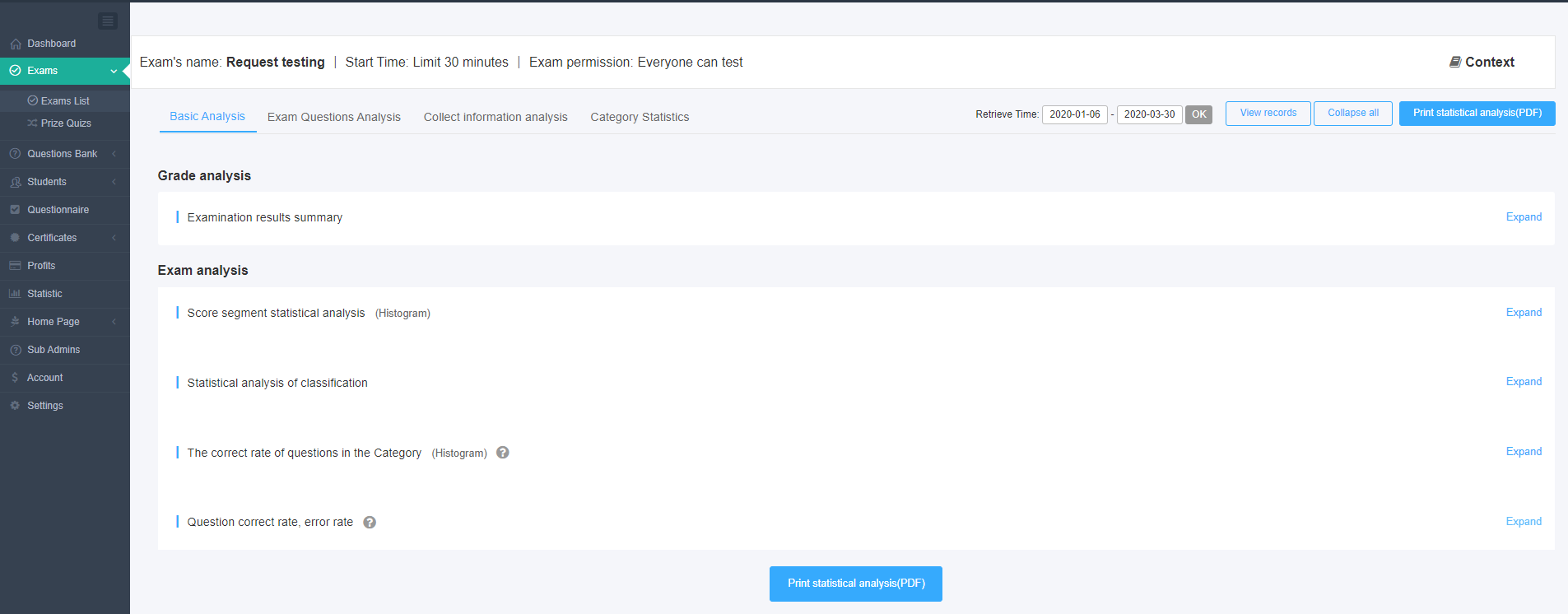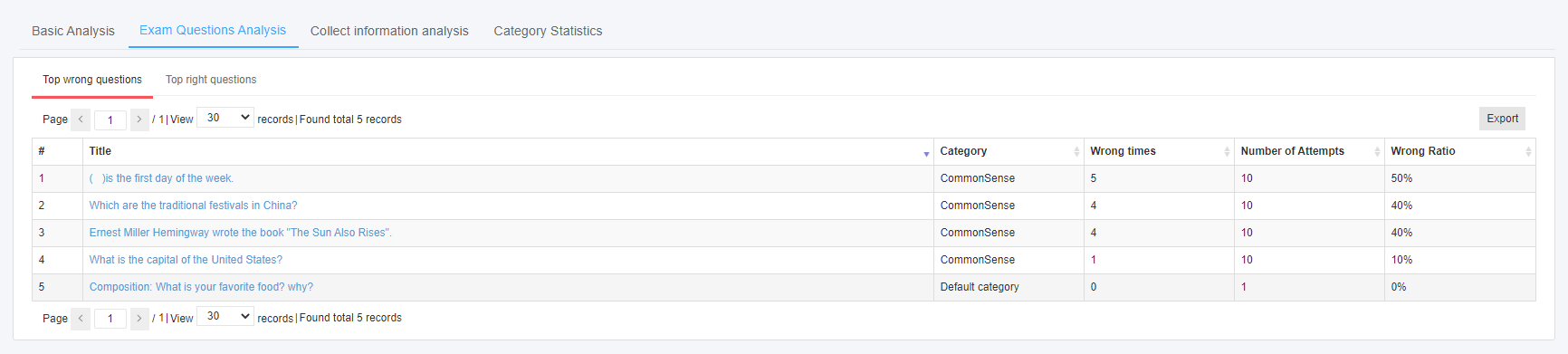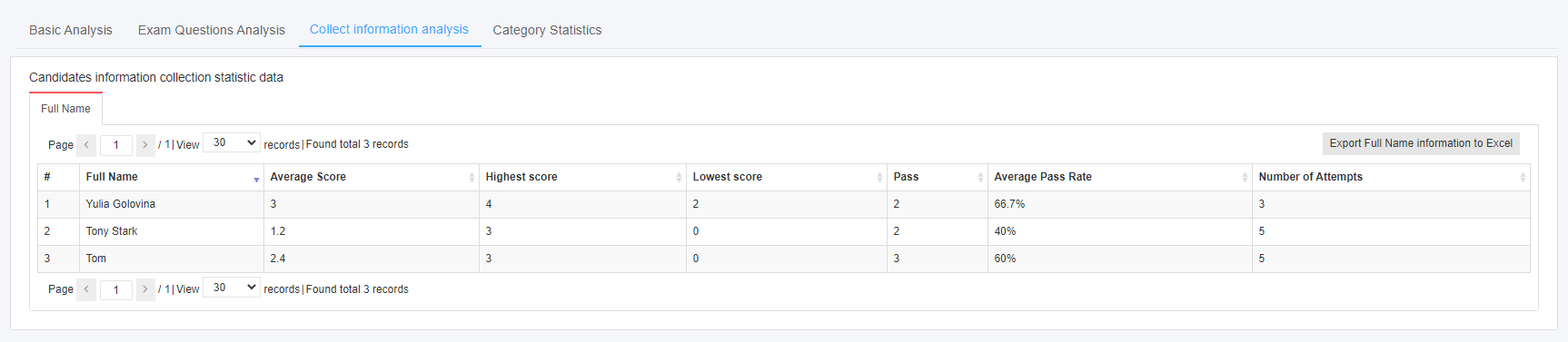# Instruction of Statistical Analysis of Test Papers¶

Click the Statistics of the exam, detailed analysis data can be seen, including Basic Analysis, Exam Questions Analysis, Collect Information Analysis, and Category Statistics.Basic Analysis

Basic analysis includes Examination results summary (pass percentage, average score, etc.), Score segment statistical analysis (Users can customize a certain score segment, and the system displays data in a histogram.), Statistical analysis of classification (Number of questions, score, correct answer rate, etc. of each category will be displayed in table format.), and Question correct rate, error rate.### Exam Questions Analysis¶

Demonstrate the ranking analysis of the error rate of the test questions.Collect Information Analysis

Analyze the candidate’s average score, lowest score, highest score, number of passes, etc. from the collected information of each candidate.Category Statistics

Show the statistics of students’ category score.